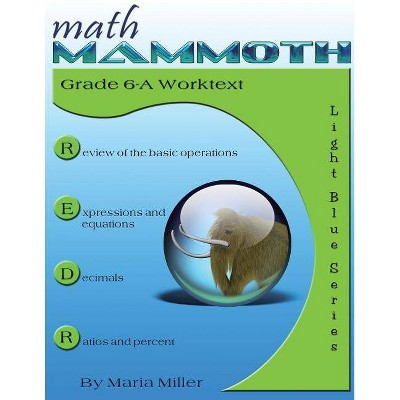# Math Mammoth Grade 6-A Worktext - by Maria Miller (Paperback)### Specifications

Number of Pages: 194

Genre: Mathematics

Sub-Genre: Arithmetic

Format: Paperback

Publisher: Math Mammoth

Age Range: 9-12 Years

Author: Maria Miller

Language: English

TCIN: 79235747
UPC: 9781942715207
Item Number (DPCI): 247-07-1619

### Description

Math Mammoth Grade 6-A Worktext is meant for the first half of grade 6. This student worktext contains both the necessary instruction and the problems & exercises (the 'text' & and the 'work'; thus a "worktext"), and is fairly self-teaching.

The main areas of study in Math Mammoth Grade 6-A are:

• review of the basic operations with whole numbers
• beginning algebra topics: expressions, equations, and inequalities
• review of all decimal arithmetic
• introduction to ratios and percent

This book starts out with a review of the four operations with whole numbers (including long division), place value, and rounding. Students are also introduced to exponents and do some problem solving.

Chapter 2 starts the study of algebra topics, delving first into expressions and equations. Students practice writing expressions in many different ways, and use properties of operations and the idea of maintaining the equality of both sides of an equation to solve simple one-step equations. We also study briefly inequalities and using two variables.

In chapter 3 we review all of decimal arithmetic, just using more decimal digits than in 5th grade. Students also practice converting measuring units.

Ratios is a new topic (chapter 4). Students are already familiar with finding fractional parts from earlier grades, and now it is time to advance that knowledge into the study of ratios, which arise naturally from dividing a quantity into many equal parts. We study such topics as rates, unit rates, equivalent ratios, and problem solving using bar models.

The goal of chapter 5 is to develop a basic understanding of percent, to see percentages as decimals, and to learn to calculate discounts.

Features

• Math Mammoth focuses on conceptual understanding. It explains the "WHY", so your children can understand the math, not just learn "HOW" to do it.
• Concepts are often explained with visual models, followed by exercises using those models. These visual models can take the place of manipulatives for many children; however, it is very easy to add corresponding manipulatives to the lessons if so desired.
• The curriculum is mastery-oriented. This means it concentrates fairly long on a topic, delving into its various aspects. This promotes conceptual understanding, as opposed to spiral curricula that often tend to jump from topic to topic too much.
• Very little teacher preparation is required.
• The curriculum has no separate teacher's manual nor is it scripted. The introduction to each chapter has some notes for the teacher concerning the material in the chapter. All the instruction is written directly to the student in the worktext, and there also exist accompanying videos where you can see Maria herself teach the material.
• After each chapter introduction, you will find a list of Internet links and resources (games, quizzes, animations, etc.) that can be used for fun, illustrations, and further practice.

The curriculum meets and exceeds the Common Core standards. This is the full-color version; in other words, the inside pages are in full color. Please note this is a student worktext and does not contain answers.

If the item details above aren’t accurate or complete, we want to know about it. Report incorrect product info.